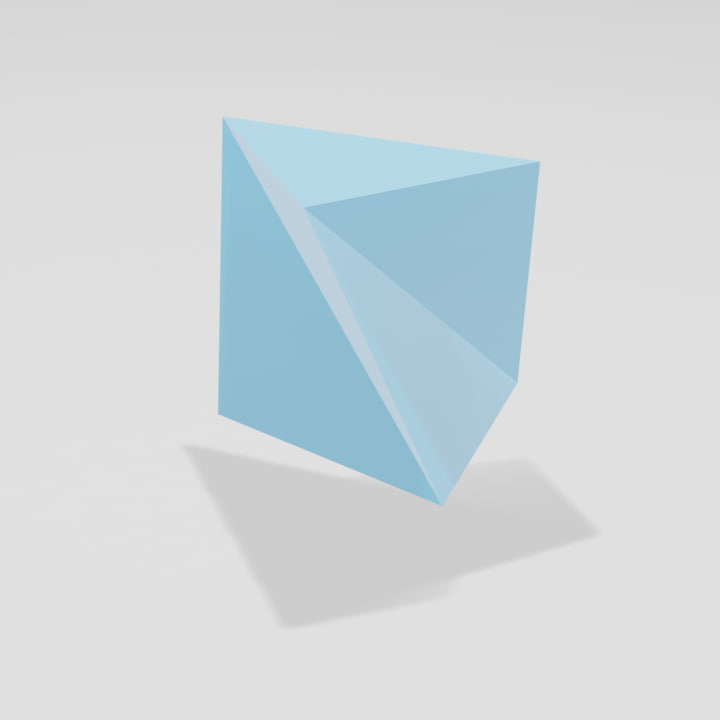# Table of Content

• 引言
• 三角形剖分的应用
• 美术馆问题
• 图形渲染问题
• 三角形剖分的算法
• 平面上的三角形算法
• 三角形剖分的优化
• 讲三角形推广到四面体的困难性
• 结论

# Document

pdf file

 Peter Giblin. “Computational geometry: algorithms and applications (2nd edn.)” In: The Mathematical Gazette 85.502 (2001), pp. 175–176.

 Steve Fisk. “A short proof of Chvátal’s Watchman Theorem”. en. In: Journal of Combinatorial Theory, Series B 24.3 (June 1978), p. 374.

 V Chvátal. “A combinatorial theorem in plane geometry”. en. In: Journal of Combinatorial Theory, Series B 18.1 (Feb. 1975), pp. 39–41.

 Joseph O’Rourke. Art gallery theorems and algorithms. en. The International series of monographs on computer science 3. New York: Oxford University Press, 1987.

 Donald Hearn, M Pauline Baker, et al. Computer graphics with OpenGL. Upper Saddle River, NJ: Pearson Prentice Hall, 2004.

 Michael R. Garey et al. “Triangulating a simple polygon”. en. In: Information Processing Letters 7.4 (June 1978), pp. 175–179.

 Robert E. Tarjan and Christopher J. Van Wyk. “An O(nloglogn)-Time Algorithm for Triangulating a Simple Polygon”. en. In: SIAM J. Comput. 17.1 (Feb. 1988), pp. 143–178.

 Kenneth L. Clarkson, Robert E. Tarjan, and Christopher J. Van Wyk. “A fast las vegas algorithm for triangu- lating a simple polygon”. en. In: Discrete Comput Geom 4.5 (Oct. 1989), pp. 423–432.

 Bernard Chazelle. “Triangulating a simple polygon in linear time”. en. In: Discrete Comput Geom 6.3 (Sept. 1991), pp. 485–524.

 William J Schroeder, Jonathan A Zarge, William E Lorensen, et al. “Decimation of triangle meshes.” In: Siggraph. Vol. 92. 1992, pp. 65–70.

 Boris Delaunay et al. “Sur la sphere vide”. In: Izv. Akad. Nauk SSSR, Otdelenie Matematicheskii i Estestvennyka Nauk 7.793-800 (1934), pp. 1–2.

 Der-Tsai Lee and Bruce J Schachter. “Two algorithms for constructing a Delaunay triangulation”. In: Interna- tional Journal of Computer & Information Sciences 9.3 (1980), pp. 219–242.

 Jim Ruppert and Raimund Seidel. “On the difficulty of triangulating three-dimensional Nonconvex Polyhedra”. en. In: Discrete Comput Geom 7.3 (Mar. 1992), pp. 227–253.

 F. Bagemihl. “On Indecomposable Polyhedra”. en. In: The American Mathematical Monthly 55.7 (Aug. 1948), pp. 411–413.

 Cristopher Moore and Stephan Mertens. The nature of computation. OCLC: ocn180753706. Oxford [England] ; New York: Oxford University Press, 2011.

# PS